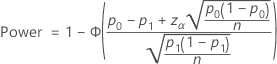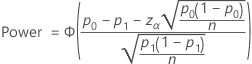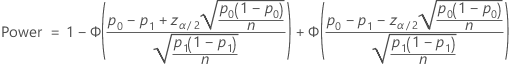# Methods and formulas for Power and Sample Size for 1 Proportion

Select the method or formula of your choice.

## Calculating power

### One-sided power (H1: p > p0)### One-sided power (H1: p < p0 )### Two-sided power (H1: p ≠ p0 )### Notation

TermDescription
p true population proportion
Φcumulative distribution function of the standard normal distribution
p 0 hypothesized proportion
p 1 comparison proportion
zα one-sided critical value (upper α point of the standard normal distribution)
z α/2 two-sided critical value (upper α/2 point of the standard normal distribution)
n sample size

## Calculating sample size and comparison proportion

If you provide values for power and sample size, Minitab calculates the value of the comparison proportion. If you provide values for power and the comparison proportion, Minitab calculates the value of the sample size.

For these two cases, Minitab uses an iterative algorithm with the power equation. At each iteration, Minitab evaluates the power for a trial sample size or trial proportion value, and stops when it reaches the values you specify.

### Target power and actual power

When Minitab calculates sample size, it may find that no integer value of sample size yields your target power. In such cases, Minitab displays the target value for power alongside the actual power, which is a value corresponding to an integer sample size, and which is nearest to, yet greater than, the target value.

By using this site you agree to the use of cookies for analytics and personalized content.  Read our policy